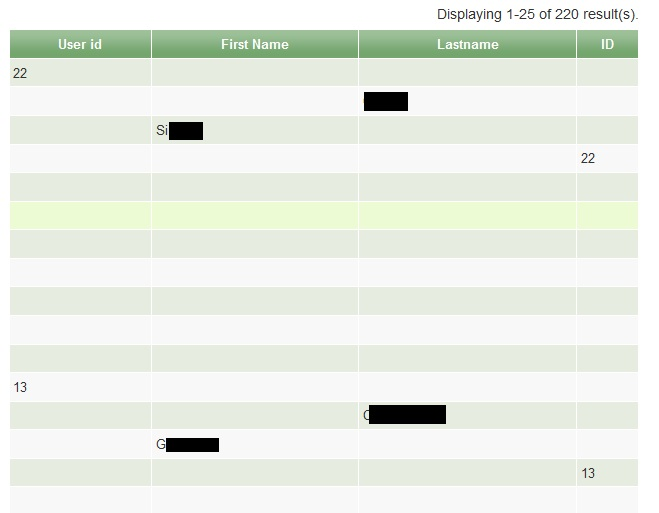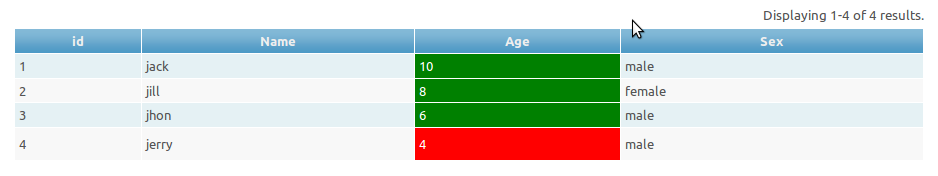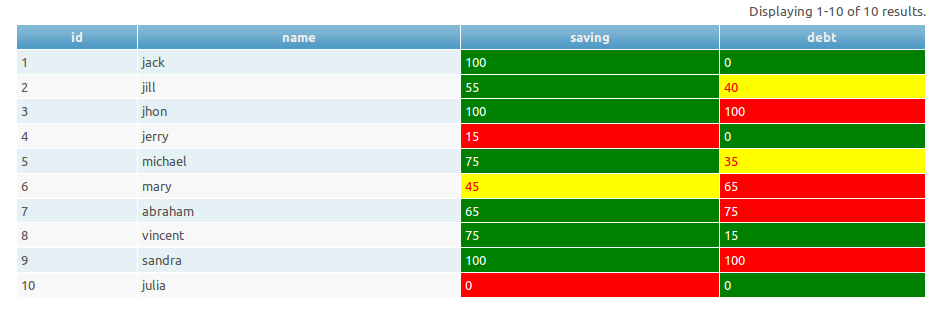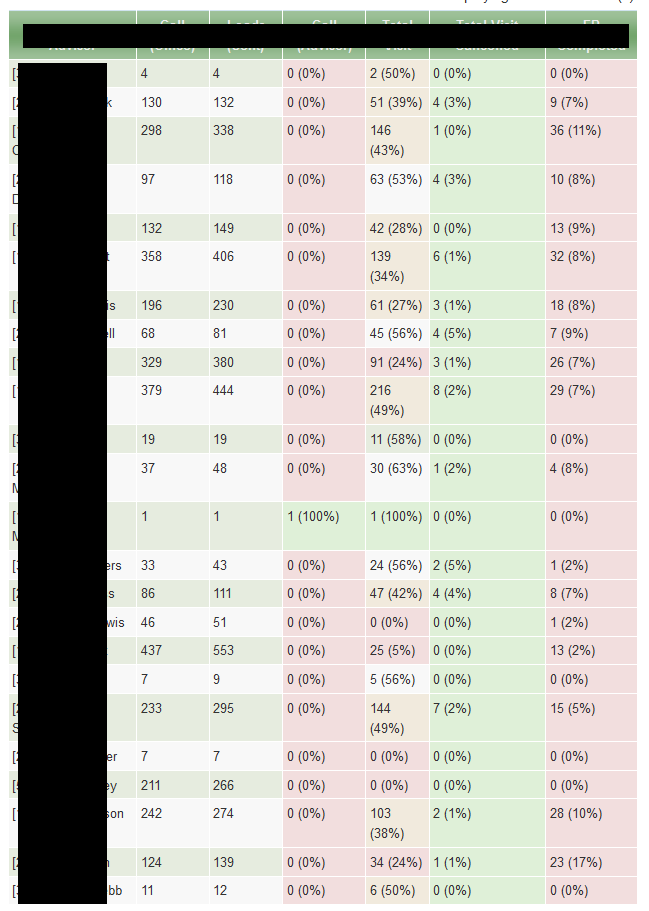# How: Yii Cgridview From Php Array?

Hi,

Any tips (or a mind reading full code snipet!) that populates a nice sortable Yii datagrid by using data from a php array?

Dear Friend

Just copy the following in a view.

``````

<?php

\$arr=array(

array('id'=>1,'name'=>"jack","age"=>10,"sex"=>"male"),

array('id'=>2,'name'=>"jill","age"=>8,"sex"=>"female"),

array('id'=>3,'name'=>"jhon","age"=>6,"sex"=>"male"),

array('id'=>4,'name'=>"jerry","age"=>4,"sex"=>"male"),

);

\$dataProvider=new CArrayDataProvider(\$arr,array(

'sort'=>array('attributes'=>array('id','name','age','sex'),),

'pagination'=>array('pageSize'=>2)

));

?>

<?php \$this->widget('zii.widgets.grid.CGridView', array(

'id'=>'post-grid',

'dataProvider'=>\$dataProvider,

'columns'=>array(

array(

'name'=>'id',

'value'=>'\$data["id"]',

),

array(

'name'=>'name',

'value'=>'\$data["name"]',

),

array(

'name'=>'age',

'value'=>'\$data["age"]',

),

array(

'name'=>'sex',

'value'=>'\$data["sex"]',

),

),

)); ?>

``````

Regards.

Hi thanks guys, will take a look.

My data comes from join two tables in SQL code, then returning it via Yii queryAll() which gives me an array, then im spitting it out into a html table with additional calculations in the coluns, so am trying to put it back into an array (not a html table) so that i can use the data in a nice sortable Yii datagrid…

i’m re-bulding the array as follows with extra bits :

``````

\$items = array();

}

``````

Using Seenivasans code, it does try to poulate the datagid, the values are in the right colums, but theres huge gaps… this is due to my array structure right? ive got a multidimensional one…

3779im getting confused now!

My standard way of making a html table works perfectly, but its static… also, is slecting DISTINCT on each user…

Dear Friend

Kindly check the following.

``````

\$items = array();

\$item=array();

\$items[]=\$item;

}

\$dataProvider=new CArrayDataProvider(\$items);

``````

Then create the Grid as I have done.

Regards.

Amazeballs!

Thank you, now im just going to get it to sort, and then to paginate more than 10…

I own you a beer.

How can i add an upper and lower limit? im changing the row colour based on a value in a cell… works fine, but i need to add limits as the last one takes precidence…

``````
'rowCssClassExpression'=>'\$data["adviser_total_visit_percent"] >= 100 ? "success ". (\$row%2 ? "even" : "odd") : (\$row%2 ? "even" : "odd")',

``````

so for example, i want warning to show, but error takes over, so want to add somehtng like the following >-50 || <= 75 (which is wrong i know)

``````
'rowCssClassExpression'=>'\$data["adviser_total_called_percent"] = 100 ? "success ". (\$row%2 ? "even" : "odd") : (\$row%2 ? "even" : "odd")',

'rowCssClassExpression'=>'\$data["adviser_total_called_percent"] >-50 || <= 75 ? "warning ". (\$row%2 ? "even" : "odd") : (\$row%2 ? "even" : "odd")',

'rowCssClassExpression'=>'\$data["adviser_total_called_percent"] <= 50 ? "error ". (\$row%2 ? "even" : "odd") : (\$row%2 ? "even" : "odd")',

``````

I never use shorthand.

Dear Friend

CComponent::evaluateExpression evaluates php strings or uses function declared in other places.

Consider that I have this column.

``````

array(

'name'=>'profile.age',

'value'=>'\$data["profile"]["age"]',

),

``````

Now I want to add class major if the age is >=18 OR class minor if age <18.

Now I am going to declare the function in the controller.

``````

public function setCellCss(\$row,\$data) //\$row and \$data are available in CGridColum during evaluation.

{

if(\$data["profile"]["age"]>=18)

return "major";

if(\$data["profile"]["age"]<18)

return "minor";

}

``````

\$row and \$data are available in CGridColum during evaluation. Here I have used \$data only.

Here you can also make use of \$row. Then you can make lot of customizations inside the function.

Now our grid column looks like this.

``````

array(

'name'=>'profile.age',

'value'=>'\$data["profile"]["age"]',

'cssClassExpression'=>array(\$this,"setCellCss"),

),

``````

Regards.

Ah, thats really great,

I can now get the cells to change their class using your function, but i’m getting full rows highlighted (im getting bracket blindness now, i’ve started off clean and basic, now im adding more and more brackets to my logic, im going bracket crazy hereI think im going mad!).

I need to get each cell to change individually, at the moment every cell in the row is changing…

I outputted the values of the \$data and they are correct, so my logic is wrong… but I need a fresh pair of eyes to spot the mistake!

you’ll see on the attachement that some cells with a value of ‘0’ are yellow or red - but should be red!

``````

// Select Colours

|| ((\$data["plans_total_completed_percent"]<50) && (\$data["plans_total_completed_percent"]>25))

)

return "warning ";

|| ((\$data["plans_total_completed_percent"]<=25) && (\$data["plans_total_completed_percent"]>=0))

)

return "error ";

``````

[attachement removed]

Dear Friend

Here is one example of using array in CArrayDataProvider.

``````

\$arr=array(

array('id'=>1,'profile'=>array('name'=>'jack','age'=>10,'sex'=>'male')),

array('id'=>2,'profile'=>array('name'=>'jill','age'=>8,'sex'=>'female')),

array('id'=>3,'profile'=>array('name'=>'jhon','age'=>6,'sex'=>'male')),

array('id'=>4,'profile'=>array('name'=>'jerry','age'=>4,'sex'=>'male')),

);

?>

<?php \$this->widget('zii.widgets.grid.CGridView', array(

'id'=>'customer-grid',

'dataProvider'=>new CArrayDataProvider(\$arr,array(

'sort'=>array(

'attributes'=>array('id','profile.name','profile.age','profile.sex')

),

'pagination'=>array('pageSize'=>10)

)),

'columns'=>array(

'id',

array(

'name'=>'profile.name',

'value'=>'\$data["profile"]["name"]'

),

array(

'name'=>'profile.age',

'value'=>'\$data["profile"]["age"]',

'cssClassExpression'=>array(\$this,"setCellCss"),//here we are setting the dynamic css.

),

array(

'name'=>'profile.sex',

'value'=>'\$data["profile"]["sex"]'

),

),

));

?>

``````

Controller.

``````

public function setCellCss(\$row,\$data)

{

if(\$data["profile"]["age"]>4)

return "big";           //css:background:green;color:white;

if(\$data["profile"]["age"]<=4)

return "small";         //css:background:red;color:white;

}

``````

This is the screenshot from my localhost.

3798Regards.

If you now add another condition, so change cell to BLUE if less than 2? the cell will still stay red…

``````

return "warning";

return "error";

``````

which is why I was adding AND logic… but getting tied up in it all!``````

/* Change colour of cell based on value */

public function setCellCss(\$row,\$data) //\$row and \$data are available in CGridColum during evaluation.

{

//Testing

\$d = \$data["plans_total_completed_percent"];

// Select Colours

|| (\$data["plans_total_completed_percent"]>50))

{

return "success";

}

|| (\$data["plans_total_completed_percent"]<50) && (\$data["plans_total_completed_percent"]>=25))

{

return "warning";

}

|| (\$data["plans_total_completed_percent"]<25) && (\$data["plans_total_completed_percent"]>=0))

{

return "error";

}

}

``````

That is still making it look as if the rows are select, so even if a value = 0, its yellow, but should be red…

Dear Friend

Let us see what happens when we reverse the logic.

``````

public function setCellCss(\$row,\$data)

{

|| (\$data["plans_total_completed_percent"]<25) && (\$data["plans_total_completed_percent"]>=0))

{

return "error";

}

|| (\$data["plans_total_completed_percent"]<50) && (\$data["plans_total_completed_percent"]>=25))

{

return "warning";

}

else

{

return "success";

}

}

``````

All red my friend… see attached… i did try that before actually…

[attachment removed]

couldit be something to do with using \$data and not \$row? clutching at straws now!

Dear Friend

This is my stupidity .I failed to guess that you are using the same function in three columns.

It is not possible to do that. The logic derived from one column is applied to other columns also.

Declaring separate function in controller for each column will make the things simpler.

The following is one workaround to use a single function for all the columns.

You have just given me the print_r output of your array source.

I struggled to convert it to array and miserably failed in that.(need a beer right now).

Then I created a scenario to simulate your one.

``````

\$arr=array(

array('id'=>1,'name'=>'jack','saving'=>100,'debt'=>0),

array('id'=>2,'name'=>'jill','saving'=>55,'debt'=>40),

array('id'=>3,'name'=>'jhon','saving'=>100,'debt'=>100),

array('id'=>4,'name'=>'jerry','saving'=>15,'debt'=>0),

array('id'=>5,'name'=>'michael','saving'=>75,'debt'=>35),

array('id'=>6,'name'=>'mary','saving'=>45,'debt'=>65),

array('id'=>7,'name'=>'abraham','saving'=>65,'debt'=>75),

array('id'=>8,'name'=>'vincent','saving'=>75,'debt'=>15),

array('id'=>9,'name'=>'sandra','saving'=>100,'debt'=>100),

array('id'=>10,'name'=>'julia','saving'=>0,'debt'=>0),

);

?>

``````

css:error(saving<25 and debt >50)

css:warning(saving 25-50 and debt 50-25)

css:ok(saving>50 debt<25)

Controller method

``````

public function setCellCss(\$row,\$data,\$object) //we have used the third parameter here.

{

if(\$object->name=="saving")

{

if(\$data["saving"]>=50)

return "ok";

if(\$data["saving"]<50 && \$data["saving"]>=25)

return "warning";

else return "error";

}

if(\$object->name=="debt")

{

if(\$data["debt"]>=50)

return "error";

if(\$data["debt"]<50 && \$data["debt"]>=25)

return "warning";

else return "ok";

}

}

``````

The \$object here refers to the column itself( instance of CDataColumn).

In above method to identify the column we have user name.We can use header, footer or id ( id would be the form ofcustomer-grid_c2 or customer-grid_c3; 0 based index).

Grid

``````

\$this->widget('zii.widgets.grid.CGridView', array(

'id'=>'customer-grid',

'dataProvider'=>new CArrayDataProvider(\$arr,array(

'sort'=>array(

'attributes'=>array('id','name','saving','debt')

),

'pagination'=>array('pageSize'=>10)

)),

'columns'=>array(

'id',

array(

'name'=>'name',

),

array(

'name'=>'saving',

'cssClassExpression'=>array(\$this,"setCellCss"),

),

array(

'name'=>'debt',

'cssClassExpression'=>array(\$this,"setCellCss"),

),

),

));

``````

The following is the screenshot.

3808Regards.

I can assure you, it is I that is stupid, you are simply a genius! lol

Great stuff! So it was after all applying to the row, due to the way it was coded, the \$object bit is perfect, ah ha!

I’ve used the revised logic function and have changed it to suit my needs, and it works!!!!! code and screen shot below!``````

public function setCellCss2(\$row,\$data,\$object) //we have used the third parameter here.

{

{

return "success";

return "warning";

return "error";

else return "no-class";

}

if(\$object->name=="total_visit")

{

return "success";

return "warning";

return "error";

else return "no-class";

}

if(\$object->name=="total_visit_cancelled")

{

return "success";

return "warning";

return "error";

else return "no-class";

}

if(\$object->name=="total_plans_completed")

{

if(\$data["plans_total_completed_percent"]>=100)

return "success";

if(\$data["plans_total_completed_percent"]<=50 && \$data["plans_total_completed_percent"]>=25)

return "warning";

if(\$data["plans_total_completed_percent"]<25 && \$data["plans_total_completed_percent"]>=0)

return "error";

else return "no-class";

}

}

``````

3812I will now go ask Bono from U2 to write a song titled “seenivasan”, so watching out for that in iTunesThank you very much seenivasan, i’ve also now got the sorting working too:

``````

\$dataProvider=new CArrayDataProvider(\$items, array(

'id'=>'user',

'sort'=>array(

'attributes'=>array(

),

),

'pagination'=>array(

'pageSize'=>25,

),

));

``````